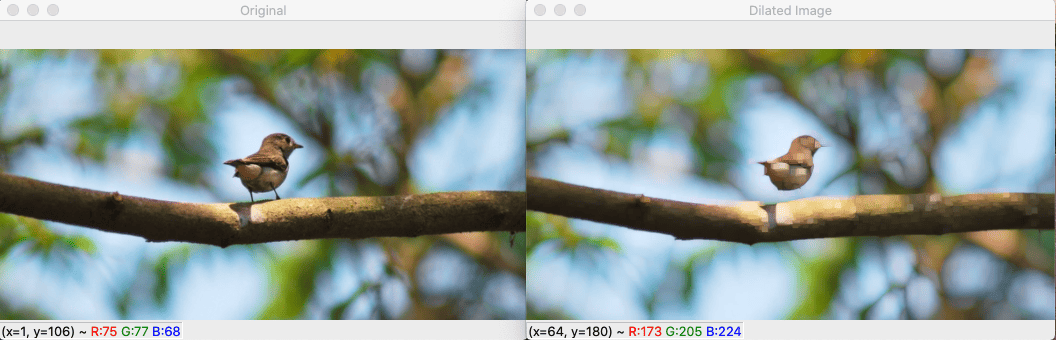# Python cv2 dilate: The Complete Guide

1
520Dilation and Erosion are two primary image operations in mathematical Morphology. Dilation is applied to binary images. The main effect of the dilation on a binary image is to continuously increase the boundaries of regions of foreground pixels (for example, white pixels, typically). Thus areas of foreground pixels expand in size while holes within those regions become smaller.

## Python cv2 dilate

The cv2.dilate() is an OpenCV function in Python that applies a morphological filter to images. The cv2.dilate() method takes two inputs, of which one is our input image; the second is called the structuring element or kernel, which decides the nature of the operation. Image dilation Increases the object area.

See the following syntax of cv2.dilate() method.

### Syntax

```cv2.dilate(src, kernel[, anchor[, iterations[, borderType[, borderValue]]]])
```

### Parameters

The dilate() function takes the following parameters.

1. image: It is a required parameter and an original image on which we need to perform dilation.
2. kernel: The required parameter is the matrix with which the image is convolved.
3. dst: It is the output image of the same size and type as image src.
4. anchor: It is a variable of type integer representing the anchor point, and its default value Point is (-1, -1), which means that the anchor is at the kernel center.
5. borderType: It depicts what kind of border to be added. Flags like cv2 define it.BORDER_CONSTANTcv2.BORDER_REFLECT, etc.
6. iterations: It is an optional parameter that takes several iterations.
7. borderValue: It is border value in case of a constant border.

The dilation increases the white region in the image or the size of the foreground object increases.

### Example

```# app.py

import numpy as np
import cv2

cv2.imshow('Original', img)

kernel = np.ones((5, 5), 'uint8')

dilate_img = cv2.dilate(img, kernel, iterations=1)
cv2.imshow('Dilated Image', dilate_img)
cv2.waitKey(0)
cv2.destroyAllWindows()
```

#### OutputIn this example, first, we have imported cv2 and numpy modules.

In the next step, we have defined a kernel for dilation. We used the np.ones() function to determine the kernel matrix. The kernel is a set of coordinates, also known as a structuring element.

Then we used the cv2.dilate() function and passed the three arguments: image, kernel, and iterations. We take iterations = 1 for this example.

In the final steps, we have displayed a dilated image.

Image dilation is helpful because if you have performed Erosion, then Erosion already removed the white noises, but it also contracts our object. So we dilate it. They won’t come back since the noise is gone, but our object area increases. Dilation helps join broken parts of an object.

#### 1 Comment

1.Kristina

Hi,
I would love to write code in Python for my Geometry class students.
We can review translation, reflection, dilation and rotation if we have the code. Please help with this?

This site uses Akismet to reduce spam. Learn how your comment data is processed.